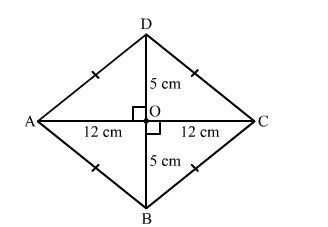# Find the length of each side of a rhombus whose diagonals are 24 cm and 10 cm long.Question:

Find the length of each side of a rhombus whose diagonals are 24 cm and 10 cm long.

Solution:Suppose ABCD is a rhombus.
We know that the digonals of a rhombus perpendicularly bisect each other.
∴ ∠AOB = 900, AO = 12 cm and BO = 5 cm
Now, In right triangle AOB
By using Pythagoras theorem we have
AB2 = AO2 + BO2
= 122 + 52
= 144 + 25
= 169
∴AB2 = 169
⇒ AB = 13 cm
Since, all the sides of a rhombus are equal.
Hence, AB = BC = CD = DA = 13 cm